The OpenFOAM Foundation
BSpline Class Reference

An implementation of B-splines. More...

Inheritance diagram for BSpline: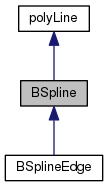[legend]
Collaboration diagram for BSpline: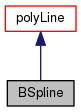[legend]

## Public Member Functions

BSpline (const pointField &knots, const bool notImplementedClosed=false)
Construct from components. More...

point position (const scalar lambda) const
Return the point position corresponding to the curve parameter. More...

point position (const label segment, const scalar lambda) const
Return the point position corresponding to the local parameter. More...

scalar length () const
Return the length of the curve. More...Public Member Functions inherited from polyLine
polyLine (const pointField &, const bool notImplementedClosed=false)
Construct from components. More...

const pointFieldpoints () const

label nSegments () const
Return the number of line segments. More...

point position (const scalar) const
Return the point position corresponding to the curve parameter. More...

point position (const label segment, const scalar) const
Return the point position corresponding to the local parameter. More...

scalar length () const
Return the length of the curve. More...Protected Member Functions inherited from polyLine
void calcParam ()
Precalculate the rational cumulative parameter value. More...

label localParameter (scalar &lambda) const
Return the line segment and the local parameter [0..1]. More...Protected Attributes inherited from polyLine
pointField points_
The control points or ends of each segments. More...

scalar lineLength_
The real line length. More...

scalarList param_
The rational (0-1) cumulative parameter value for each point. More...

## Detailed Description

An implementation of B-splines.

In this implementation, the end tangents are created automatically by reflection.

In matrix form, the local interpolation on the interval t=[0..1] is described as follows:

```P(t) = 1/6 * [ t^3 t^2 t 1 ] * [ -1  3 -3  1 ] * [ P-1 ]
[  3 -6  3  0 ]   [ P0 ]
[ -3  0  3  0 ]   [ P1 ]
[  1  4  1  0 ]   [ P2 ]
```

Where P-1 and P2 represent the neighbouring points or the extrapolated end points. Simple reflection is used to automatically create the end points.

The spline is discretized based on the chord length of the individual segments. In rare cases (sections with very high curvatures), the resulting distribution may be sub-optimal.

A future implementation could also handle closed splines.

CatmullRomSpline
Source files

Definition at line 73 of file BSpline.H.

## Constructor & Destructor Documentation

 BSpline ( const pointField & knots, const bool notImplementedClosed = `false` )

Construct from components.

Definition at line 32 of file BSpline.C.

## Member Function Documentation

 Foam::point position ( const scalar lambda ) const

Return the point position corresponding to the curve parameter.

0 <= lambda <= 1

Definition at line 43 of file BSpline.C.

Referenced by BSplineEdge::position().

Here is the call graph for this function: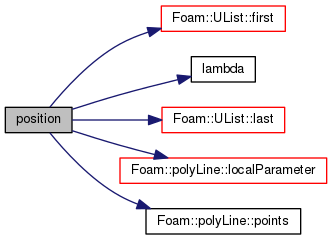Here is the caller graph for this function: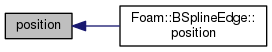Foam::point position ( const label segment, const scalar lambda ) const

Return the point position corresponding to the local parameter.

0 <= lambda <= 1 on the given segment

Definition at line 62 of file BSpline.C.

Here is the call graph for this function: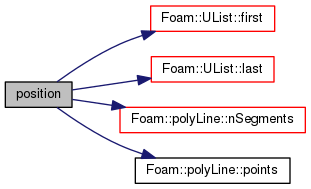Foam::scalar length ( ) const

Return the length of the curve.

Definition at line 133 of file BSpline.C.

References NotImplemented.

Referenced by BSplineEdge::length().

Here is the caller graph for this function: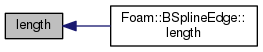The documentation for this class was generated from the following files: